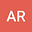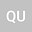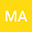F-Expansion Method and Its Application for Finding Exact Analytical Solutions of Fractional order RLW Equation
•••• +1
• Attia Rani,
• Qazi Mahmood Ul-Hassan,
Attia Rani
University of Wah
Author ProfileQazi Mahmood Ul-Hassan
University of Wah
Author ProfileUniversity of Wah
Author ProfileUniversity of Gujrat
Author Profile## Abstract

Exact nonlinear partial differential equation solutions are critical for describing new complex characteristics in a variety of fields of applied science. The aim of this research is to use the F-expansion method to find the generalized solitary wave solution of the regularized long wave (RLW) equation of fractional order. Fractional partial differential equations can also be transformed into ordinary differential equations using fractional complex transformation and the properties of the modified Riemann–Liouville fractional-order operator. Because of the chain rule and the derivative of composite functions, nonlinear fractional differential equations (NLFDEs) can be converted to ordinary differential equations. We have investigated various set of explicit solutions with some free parameters using this approach. The solitary wave solutions are derived from the moving wave solutions when the parameters are set to special values. Our findings show that this approach is a very active and straightforward way of formulating exact solutions to nonlinear evolution equations that arise in mathematical physics and engineering. It is anticipated that this research will provide insight and knowledge into the implementation of novel methods for solving wave equations.

#### Peer review status:UNDER REVIEW

10 Jun 2021Submitted to Mathematical Methods in the Applied Sciences
11 Jun 2021Assigned to Editor
11 Jun 2021Submission Checks Completed
12 Jun 2021Reviewer(s) Assigned